# Prime and composite numbers Prime factors

## The definition of a Prime number

Definition: a Natural number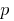is called simpleif it has only two positive divisors — the number itselfand 1.

2, 3, 5, 7, 11, 13, 17, 19, 23, 29, 31, 37, 41, 43, ... — the Prime numbers.

Prime numbers are infinitely many.

## The definition of composite numbers

Definition: a Natural number is called compositeif it has more than two positive divisors.

6, 15, 130, ... are composite numbers.

1 is neither Prime number nor composite.

## Properties of Prime factors of natural numbers

1. Any natural number (greater than unity) or is divided by this Prime number, or is coprime with it.
2. If the product of several factors is divisible by a Prime number, then at least one of the factors is divisible by.
3. The smallest Prime divisor of a composite number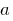not to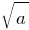.

## The main theorem of the theory of divisibility

Theorem: Any natural number greater than unity, can be decomposed into the product of primes, and this decomposition is unique up the order of the multiplicands.where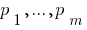are all Prime numbers.

## The theorem on division with Stacey

Theorem: For any pair of numbersand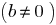there is, and only one pair of integers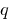and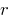such that, where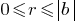(is the incomplete quotient ofat,— moduloon).

Tags:
Chapter:
Versions in other languages:
Share with friends: